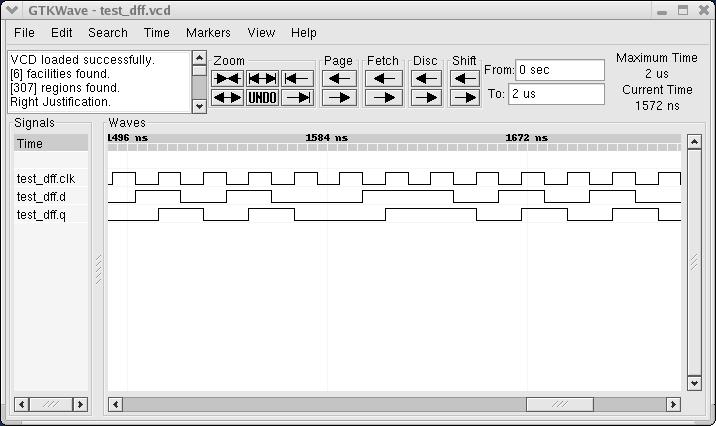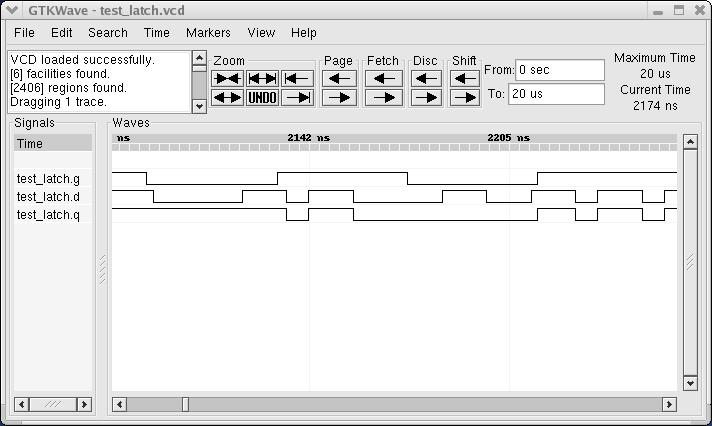## Introduction

On this page you will find a number of MyHDL descriptions of flip-flops and latches.

Typically, you wouldn't describe flip-flops and latches as individual modules. Rather, they can be inferred from higher-level RTL description by a synthesis tool. However, as these circuits are small and widely known, they are well suited to explain basic MyHDL usage and to compare MyHDL with other solutions.

## D flip-flop

### Specification

The basic D flip-flop is a sequential device that transfers the value of the `d` input to the `q` output on every rising edge of the clock `clk`.

### Description

Here is the description of a D flip-flop in MyHDL:

```from myhdl import *

@block
def dff(q, d, clk):

@always(clk.posedge)
def logic():
q.next = d

return logic
```

### Simulation

Let's add a small test bench to simulate the design:

```from random import randrange

@block
def test_dff():

q, d, clk = [Signal(bool(0)) for i in range(3)]

dff_inst = dff(q, d, clk)

@always(delay(10))
def clkgen():
clk.next = not clk

@always(clk.negedge)
def stimulus():
d.next = randrange(2)

return dff_inst, clkgen, stimulus

def simulate(timesteps):
simInst = test_dff()
simInst.config_sim(trace=True, tracebackup=False)
simInst.run_sim(timesteps, quiet=0)

simulate(2000)
```

Function `test_dff` creates an instance of the D flip-flop, and adds a clock generator and a random stimulus generator around it.

Function `simulate` simulates the test bench. Note how the MyHDL function `traceSignals` is used to create the test bench instance (instead of calling `test_dff` directly). As a result, a signal trace file will be created during simulation. This file can be inspecting in a waveform viewer. As a verification method, inspecting waveforms has its drawbacks, but it can be very effective to debug small designs.

Here's a screen shot of the simulation waveforms:### Automatic conversion to Verilog or VHDL

With MyHDL's `convert` function, a D flip-flop instance can be converted to Verilog or VHDL code:

```def convert():
q, d, clk = [Signal(bool(0)) for i in range(3)]
convInst = dff(q, d, clk)  #, 'Verilog')
convInst.convert(hdl='Verilog')
convInst.convert(hdl='VHDL')

convert()
```

This is the resulting Verilog code:

```module dff (
q,
d,
clk
);

output q;
reg q;
input d;
input clk;

always @(posedge clk) begin: DFF_LOGIC
q <= d;
end

endmodule
```

## D flip-flop with asynchronous reset

### Specification

One of the most useful sequential building blocks is a D flip-flop with an additional asynchronous reset pin. When the reset is not active, it operates as a basic D flip-flop as in the previous section. When the reset pin is active, the output is held to zero. Typically, the reset pin is active low.

### Description

Here is the description:

```from myhdl import *

@block
def dffa(q, d, clk, rst):

@always_seq(clk.posedge, reset=rst)
def logic():
if rst == 0:    # nRst ?  or use active high ?
q.next = 0
else:
q.next = d

return logic
```

### Simulation

Here is a test bench for the design:

```from random import randrange

@block
def test_dffa():

q, d, clk = [Signal(bool(0)) for i in range(3)]
rst = ResetSignal(val=1, active=0, isasync=True)

dffa_inst01 = dffa(q, d, clk, rst)

@always(delay(10))
def clkgen():
clk.next = not clk

@always(clk.negedge)
def stimulus():
d.next = randrange(2)

@instance
def rstgen():
yield delay(5)
rst.next = 1
while True:
yield delay(randrange(500, 1000))
rst.next = 0
yield delay(randrange(80, 140))
rst.next = 1

return dffa_inst, clkgen, stimulus, rstgen

def simulate(timesteps):
simInst = test_dffa()
simInst.config_sim(trace=True, tracebackup=False)
simInst.run_sim(timesteps, quiet=0)

simulate(2000)
```

Compared to the test bench for the basic D flip-flop, there is an additional reset generator, that generates reset pulses at random moments and with a random duration.

Here is a screen shot of the waveforms:### Automatic conversion to Verilog or VHDL

The design can be converted to Verilog and VHDL:

```def convert():
q, d, clk = [Signal(bool(0)) for i in range(3)]
rst = ResetSignal(val=1, active=0, isasync=True)
convInst = dffa(q, d, clk, rst)
convInst.convert(hdl='Verilog')
convInst.convert(hdl='VHDL')

convert()
```

The VHDL result looks like this:

```entity dffa is
port (
q: out std_logic;
d: in std_logic;
clk: in std_logic;
rst: in std_logic
);
end entity dffa;

architecture MyHDL of dffa is
begin
DFFA_LOGIC: process (clk, rst) is
begin
if (rst = '0') then
q <= '0';
elsif rising_edge(clk) then
if (rst = '0') then
q <= '0';
else
q <= d;
end if;
end if;
end process DFFA_LOGIC;

end architecture MyHDL;
```

## Latch

### Specification

A basic latch is a sequential device with an input, and output and a control gate pin. When the gate is open, the output follows the input combinatorially. When it is closed, the output keeps its value.

### Description

The following code describes a latch:

```from myhdl import *

@block
def latch(q, d, g):

@always_comb
def logic():
if g == 1:
q.next = d

return logic
```

Note the usage of the `always_comb` decorator. This is somewhat of a misnomer. (The name comes from a similar construct in SystemVerilog). It doesn't mean the generator describes a circuit that is necessarily combinatorial, but merely that it triggers whenever one of the input signals changes.

### Simulation

Here is a test bench to simulate the latch:

```from random import randrange

@block
def test_latch():

q, d, g = [Signal(bool(0)) for i in range(3)]

latch_inst = latch(q, d, g)

@always(delay(7))
def dgen():
d.next = randrange(2)

@always(delay(41))
def ggen():
g.next = randrange(2)

return latch_inst, dgen, ggen

def simulate(timesteps):
simInst = test_latch()
simInst.config_sim(trace=True)
simInst.run_sim(timesteps, quiet=0)

simulate(2000)
```

In addition to the latch instance, the test bench creates a random data generator for the input and for the controlling gate.

Here is a screen shot of the simulation waveforms:### Automatic conversion to Verilog or VHDL

We can convert the design as follows:

```def convert():
q, d, g = [Signal(bool(0)) for i in range(3)]
convInst = latch(q, d, g)
convInst.convert(hdl='Verilog')
convInst.convert(hdl='VHDL')

convert()
```

Here is the verilog result:

```module latch (
q,
d,
g
);

output q;
reg q;
input d;
input g;

always @(g, d) begin: LATCH_LOGIC
if ((g == 1)) begin
q = d;
end
end

endmodule
```

Note that when the `convert` function converts the `always_comb` decorator, it infers which signals are used as inputs to the `always` block